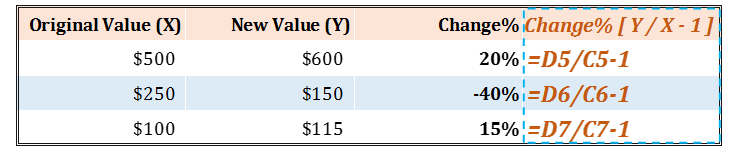# Calculating Change %By saying change %tage – we essentially mean that the percentage of increase or decrease of a number from its original value to a new value.

To calculate the increase percentage: First: get the difference (increase) between the two numbers you are comparing. Then: divide the increase by the original number and multiply the answer by 100.

If your answer is a negative number, then this is a percentage decrease.

Calculating Change % in MS Excel

Let’s understand how to calculate this in MS Excel.

Suppose we have a number ‘X’ which has changed to a new value called ‘Y’, then the change % would be calculated as under:#### 1 COMMENT

1.Joe

Hi Sir, this is really a very helpful and easy formula to calculate change%. Thanks, pls keep sharing such useful calculation tricky formulas. Thanks!!